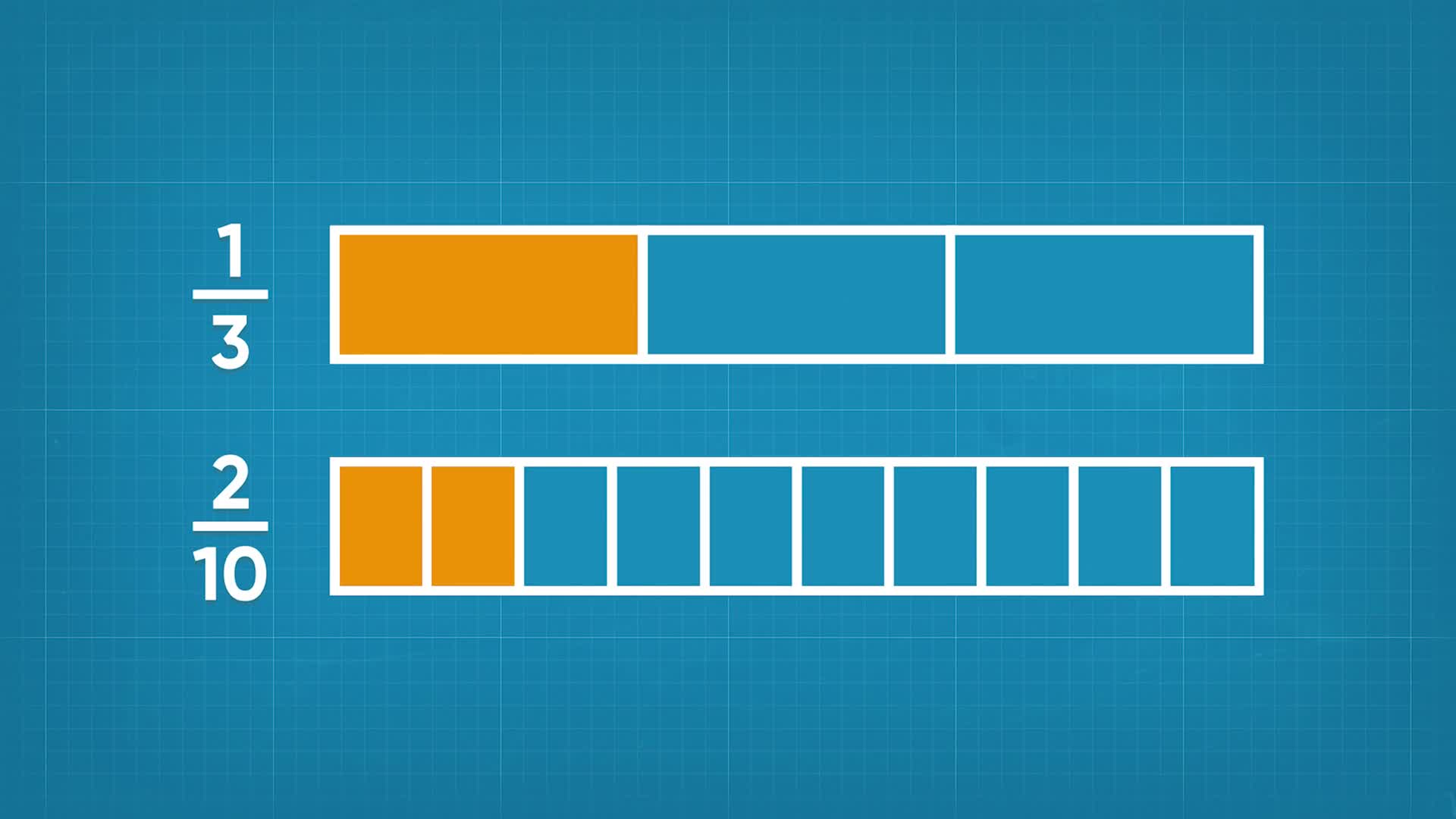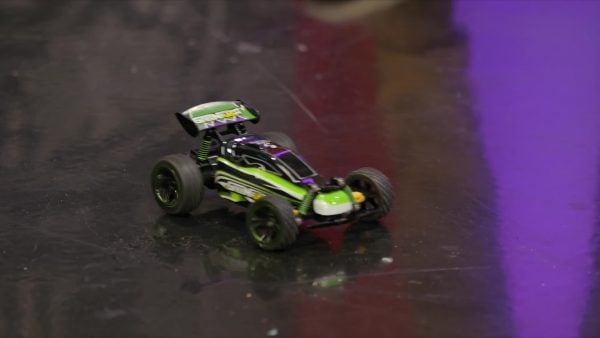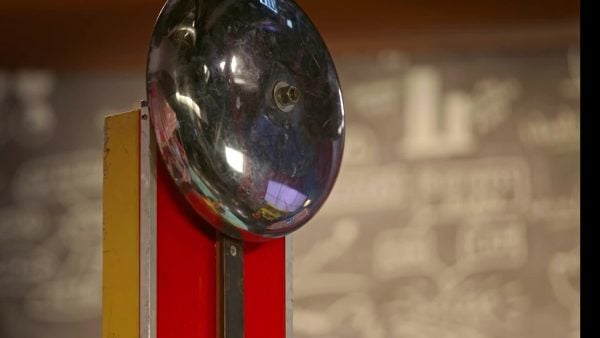Compare Non-Equivalent Fractions Math Video for Kids - Grades 3-5
1%
It was processed successfully!WHAT ARE NON-EQUIVALENT FRACTIONS?

Non-Equivalent fractions represent different fractions of a whole. You can use common denominators to compare fractions.

To better understand comparing non-equivalent fractions…

WHAT ARE NON-EQUIVALENT FRACTIONS?. Non-Equivalent fractions represent different fractions of a whole. You can use common denominators to compare fractions. To better understand comparing non-equivalent fractions…

## LET’S BREAK IT DOWN!

### BalloonsAdesina, April, and Marcos prepare balloons for a party. They have one helium tank that is [ggfrac]3/4[/ggfrac] full, and another that is [ggfrac]2/5[/ggfrac] full. Which tank is more full? The two fractions have different denominators, which makes it harder to directly compare them. One way we can compare fractions is by using a benchmark fraction. Let’s use [ggfrac]1/2[/ggfrac] as a benchmark. Since [ggfrac]1/2[/ggfrac] is equivalent to [ggfrac]2/4[/ggfrac], we can see that [ggfrac]3/4[/ggfrac] is more than [ggfrac]1/2[/ggfrac]. We also know that [ggfrac]2/5[/ggfrac] is less than [ggfrac]1/2[/ggfrac]. Then, since [ggfrac]2/5[/ggfrac] is less than [ggfrac]1/2[/ggfrac], and [ggfrac]3/4[/ggfrac] is more than [ggfrac]1/2[/ggfrac], [ggfrac]3/4[/ggfrac] is greater than [ggfrac]2/5[/ggfrac]! Now you try: Use a benchmark fraction to determine which is greater, [ggfrac]5/8[/ggfrac] or [ggfrac]3/7[/ggfrac].

Balloons Adesina, April, and Marcos prepare balloons for a party. They have one helium tank that is [ggfrac]3/4[/ggfrac] full, and another that is [ggfrac]2/5[/ggfrac] full. Which tank is more full? The two fractions have different denominators, which makes it harder to directly compare them. One way we can compare fractions is by using a benchmark fraction. Let’s use [ggfrac]1/2[/ggfrac] as a benchmark. Since [ggfrac]1/2[/ggfrac] is equivalent to [ggfrac]2/4[/ggfrac], we can see that [ggfrac]3/4[/ggfrac] is more than [ggfrac]1/2[/ggfrac]. We also know that [ggfrac]2/5[/ggfrac] is less than [ggfrac]1/2[/ggfrac]. Then, since [ggfrac]2/5[/ggfrac] is less than [ggfrac]1/2[/ggfrac], and [ggfrac]3/4[/ggfrac] is more than [ggfrac]1/2[/ggfrac], [ggfrac]3/4[/ggfrac] is greater than [ggfrac]2/5[/ggfrac]! Now you try: Use a benchmark fraction to determine which is greater, [ggfrac]5/8[/ggfrac] or [ggfrac]3/7[/ggfrac].

### Remote Control CarsApril and Marcos drive remote control cars. Both cars have the same size battery. Marcos’s car has [ggfrac]2/3[/ggfrac] of its battery power left, and April's car has [ggfrac]5/6[/ggfrac] left. Whose battery has more power left? Since both [ggfrac]2/3[/ggfrac] and [ggfrac]5/6[/ggfrac] represent more than [ggfrac]1/2[/ggfrac], we can't use the benchmark of [ggfrac]1/2[/ggfrac] to compare them. Instead, we can use common denominators. We can use our knowledge of equivalent fractions to rewrite [ggfrac]2/3[/ggfrac] and [ggfrac]5/6[/ggfrac] so that they have the same denominator. 3 x 2 = 6, so we can multiply the numerator and denominator of [ggfrac]2/3[/ggfrac] by 2 to get an equivalent fraction with a denominator of 6. [ggfrac]2x2/3x2[/ggfrac] = [ggfrac]4/6[/ggfrac]. Now we compare [ggfrac]4/6[/ggfrac] to [ggfrac]5/6[/ggfrac]. Both fractions have the same denominator, so we can compare the fractions using their numerators. 5 > 4, so [ggfrac]5/6[/ggfrac] is greater than [ggfrac]4/6[/ggfrac]. April's battery has more power left. Now you try: Use common denominators to compare [ggfrac]3/5[/ggfrac] and [ggfrac]7/10[/ggfrac].

Remote Control Cars April and Marcos drive remote control cars. Both cars have the same size battery. Marcos’s car has [ggfrac]2/3[/ggfrac] of its battery power left, and April's car has [ggfrac]5/6[/ggfrac] left. Whose battery has more power left? Since both [ggfrac]2/3[/ggfrac] and [ggfrac]5/6[/ggfrac] represent more than [ggfrac]1/2[/ggfrac], we can't use the benchmark of [ggfrac]1/2[/ggfrac] to compare them. Instead, we can use common denominators. We can use our knowledge of equivalent fractions to rewrite [ggfrac]2/3[/ggfrac] and [ggfrac]5/6[/ggfrac] so that they have the same denominator. 3 x 2 = 6, so we can multiply the numerator and denominator of [ggfrac]2/3[/ggfrac] by 2 to get an equivalent fraction with a denominator of 6. [ggfrac]2x2/3x2[/ggfrac] = [ggfrac]4/6[/ggfrac]. Now we compare [ggfrac]4/6[/ggfrac] to [ggfrac]5/6[/ggfrac]. Both fractions have the same denominator, so we can compare the fractions using their numerators. 5 > 4, so [ggfrac]5/6[/ggfrac] is greater than [ggfrac]4/6[/ggfrac]. April's battery has more power left. Now you try: Use common denominators to compare [ggfrac]3/5[/ggfrac] and [ggfrac]7/10[/ggfrac].

### CarnivalApril and Marcos are at a carnival strength challenge. They swing a mallet and a machine records the strength of the blow, up to a marker. Marcos strikes with his mallet and he scores [ggfrac]3/4[/ggfrac] of the way to the marker. April strikes with her mallet and she scores [ggfrac]4/5[/ggfrac] of the way to the marker. Who got the greater score? We can use equivalent fractions to compare. 5 is not a multiple of 4, so we need to rewrite both fractions to make the fractions have a common denominator. Some equivalent fractions of [ggfrac]3/4[/ggfrac] are [ggfrac]6/8[/ggfrac], [ggfrac]9/12[/ggfrac], [ggfrac]12/16[/ggfrac], [ggfrac]15/20[/ggfrac], and [ggfrac]18/24[/ggfrac]. Some equivalent fractions of [ggfrac]4/5[/ggfrac] are [ggfrac]8/10[/ggfrac], [ggfrac]12/15[/ggfrac], and [ggfrac]16/20[/ggfrac]. Both fractions have an equivalent fraction with a denominator of 20: [ggfrac]3/4[/ggfrac] = [ggfrac]15/20[/ggfrac] and [ggfrac]4/5[/ggfrac] = [ggfrac]16/20[/ggfrac]. Now that the fractions have the same denominator, we can compare the numerators. 16 > 15, so [ggfrac]16/20[/ggfrac] > [ggfrac]15/20[/ggfrac]. Since [ggfrac]16/20[/ggfrac] = [ggfrac]4/5[/ggfrac], and [ggfrac]15/20[/ggfrac] = [ggfrac]3/4[/ggfrac], we know that [ggfrac]4/5[/ggfrac] > [ggfrac]3/4[/ggfrac]. There is a faster way to find the common denominators than listing equivalent fractions. For each fraction, multiply the numerator and the denominator by the denominator of the other fraction. For example, to compare [ggfrac]3/4[/ggfrac] and [ggfrac]4/5[/ggfrac], multiply [ggfrac]4/5[/ggfrac] by [ggfrac]4/4[/ggfrac] to get [ggfrac]16/20[/ggfrac], and multiply [ggfrac]3/4[/ggfrac] by [ggfrac]5/5[/ggfrac] to get [ggfrac]15/20[/ggfrac]. That was faster! Now you try: Use common denominators to decide which is greater, [ggfrac]6/7[/ggfrac] or [ggfrac]4/5[/ggfrac].

Carnival April and Marcos are at a carnival strength challenge. They swing a mallet and a machine records the strength of the blow, up to a marker. Marcos strikes with his mallet and he scores [ggfrac]3/4[/ggfrac] of the way to the marker. April strikes with her mallet and she scores [ggfrac]4/5[/ggfrac] of the way to the marker. Who got the greater score? We can use equivalent fractions to compare. 5 is not a multiple of 4, so we need to rewrite both fractions to make the fractions have a common denominator. Some equivalent fractions of [ggfrac]3/4[/ggfrac] are [ggfrac]6/8[/ggfrac], [ggfrac]9/12[/ggfrac], [ggfrac]12/16[/ggfrac], [ggfrac]15/20[/ggfrac], and [ggfrac]18/24[/ggfrac]. Some equivalent fractions of [ggfrac]4/5[/ggfrac] are [ggfrac]8/10[/ggfrac], [ggfrac]12/15[/ggfrac], and [ggfrac]16/20[/ggfrac]. Both fractions have an equivalent fraction with a denominator of 20: [ggfrac]3/4[/ggfrac] = [ggfrac]15/20[/ggfrac] and [ggfrac]4/5[/ggfrac] = [ggfrac]16/20[/ggfrac]. Now that the fractions have the same denominator, we can compare the numerators. 16 > 15, so [ggfrac]16/20[/ggfrac] > [ggfrac]15/20[/ggfrac]. Since [ggfrac]16/20[/ggfrac] = [ggfrac]4/5[/ggfrac], and [ggfrac]15/20[/ggfrac] = [ggfrac]3/4[/ggfrac], we know that [ggfrac]4/5[/ggfrac] > [ggfrac]3/4[/ggfrac]. There is a faster way to find the common denominators than listing equivalent fractions. For each fraction, multiply the numerator and the denominator by the denominator of the other fraction. For example, to compare [ggfrac]3/4[/ggfrac] and [ggfrac]4/5[/ggfrac], multiply [ggfrac]4/5[/ggfrac] by [ggfrac]4/4[/ggfrac] to get [ggfrac]16/20[/ggfrac], and multiply [ggfrac]3/4[/ggfrac] by [ggfrac]5/5[/ggfrac] to get [ggfrac]15/20[/ggfrac]. That was faster! Now you try: Use common denominators to decide which is greater, [ggfrac]6/7[/ggfrac] or [ggfrac]4/5[/ggfrac].

### MarathonApril and Marcos are training for a race. April ran [ggfrac]3/5[/ggfrac] of a mile and Marcos ran [ggfrac]5/6[/ggfrac] of a mile. Who ran farther? We need to find a common denominator to compare fractions that have different denominators. Multiply the numerator and denominator of [ggfrac]3/5[/ggfrac] by 6: [ggfrac]3x6/5x6[/ggfrac] = [ggfrac]25/30[/ggfrac]. Multiply the numerator and denominator of [ggfrac]5/6[/ggfrac] by 5: [ggfrac]5x5/6x5[/ggfrac] = [ggfrac]25/30[/ggfrac]. Now that the denominators are the same, compare the numerators. Since 25 > 18, [ggfrac]25/30[/ggfrac] > [ggfrac]18/30[/ggfrac]. So[ggfrac]5/6[/ggfrac] > [ggfrac]3/5[/ggfrac]. Now you try: Which is greater, [ggfrac]2/3[/ggfrac] or [ggfrac]3/4[/ggfrac]?

Marathon April and Marcos are training for a race. April ran [ggfrac]3/5[/ggfrac] of a mile and Marcos ran [ggfrac]5/6[/ggfrac] of a mile. Who ran farther? We need to find a common denominator to compare fractions that have different denominators. Multiply the numerator and denominator of [ggfrac]3/5[/ggfrac] by 6: [ggfrac]3x6/5x6[/ggfrac] = [ggfrac]25/30[/ggfrac]. Multiply the numerator and denominator of [ggfrac]5/6[/ggfrac] by 5: [ggfrac]5x5/6x5[/ggfrac] = [ggfrac]25/30[/ggfrac]. Now that the denominators are the same, compare the numerators. Since 25 > 18, [ggfrac]25/30[/ggfrac] > [ggfrac]18/30[/ggfrac]. So[ggfrac]5/6[/ggfrac] > [ggfrac]3/5[/ggfrac]. Now you try: Which is greater, [ggfrac]2/3[/ggfrac] or [ggfrac]3/4[/ggfrac]?

## COMPARE NON-EQUIVALENT FRACTIONS VOCABULARY

Fraction
A number that represents a part of a whole.
Numerator
The top number in a fraction, which tells us how many parts have been counted.
Denominator
The bottom number in a fraction, which tells us how many parts are in the whole.
Equivalent fractions
Fractions that represent the same amount.
Non-equivalent fractions
Fractions that represent different amounts.
Benchmark fraction
A familiar fraction that we can compare other fractions to.
Having the same denominator in two fractions.

## COMPARE NON-EQUIVALENT FRACTIONS DISCUSSION QUESTIONS

### What benchmark fraction can you use to compare [ggfrac]1/3[/ggfrac] and [ggfrac]3/4[/ggfrac]? Why?

I can use [ggfrac]1/2[/ggfrac] as a benchmark fraction. [ggfrac]1/2[/ggfrac] = [ggfrac]2/4[/ggfrac], so [ggfrac]3/4[/ggfrac] is more than [ggfrac]1/2[/ggfrac]. Since [ggfrac]1/3[/ggfrac] is less than [ggfrac]1/2[/ggfrac], we know that [ggfrac]3/4[/ggfrac] is greater than [ggfrac]1/3[/ggfrac].

### Can you use [ggfrac]1/2[/ggfrac] as a benchmark fraction to compare [ggfrac]4/5[/ggfrac] and [ggfrac]6/7[/ggfrac]?

[ggfrac]1/2[/ggfrac] is not very useful because both [ggfrac]4/5[/ggfrac] and [ggfrac]6/7[/ggfrac] are greater than [ggfrac]1/2[/ggfrac].

### Why is it hard to compare two fractions that have different denominators?

Denominators tell the size of the unit, so if I have two different denominators, it is like comparing different numbers of different-sized slices.

### What can you do to compare two fractions that have different denominators, when benchmarks won't work?

I can find equivalent fractions for each fraction until I find two that have the same denominator.

### You have two lasagnas: Lasagna #1 has [ggfrac]1/2[/ggfrac] left, and lasagna #2 has [ggfrac]2/3[/ggfrac] left. How could you cut each lasagna so that both lasagnas have the same-sized slices?

I could cut the slice in the first lasagna into three equal-sized pieces, and cut each of the slices in the second lasagna into two equal-sized pieces. Now each of the lasagnas can be expressed using 6 as a denominator.
X

## Success

We’ve sent you an email with instructions how to reset your password.
Ok

## Choose Your Free Trial Period### 7 DaysContinue to Lessons### 90 DaysGet 90 days free by inviting other teachers to try it too.

Share with Teachers

## Get 90 Days Free

### By inviting 4 other teachers to try it too.

4 required

Skip, I will use a 7 days free trial

## Thank You!

Enjoy your free 90 days trial